HomeBlogArtificial IntelligenceDeep Learning Algorithm [Comprehensive Guide With Examples]

# Deep Learning Algorithm [Comprehensive Guide With Examples]

Last updated:
4th Jun, 2023
Views
17 MinsView All## Introduction

Deep Learning is a subset of machine learning, which involves algorithms inspired by the arrangement and functioning of the brain. As neurons from human brains transmit information and help in learning from the reactors in our body, similarly the deep learning algorithms run through various layers of neural networks algorithms and learn from their reactions.

In other words, Deep learning utilizes layers of neural network algorithms to discover more significant level data dependent on raw input data. The neural network algorithms discover the data patterns through a process that simulates in a manner of how a human brain works.

Neural networks help in clustering the data points from a large set of data points based upon the similarities of the features. These systems are known as Artificial Neural Networks.

As more and more data were fed to the models, deep learning algorithms proved out to be more productive and provide better results than the rest of the algorithms. Deep Learning algorithms are used for various problems like image recognition, speech recognition, fraud detection, computer vision etc.

Get Machine Learning Certification from the World’s top Universities. Earn Masters, Executive PGP, or Advanced Certificate Programs to fast-track your career.

## Components of Neural Network

1. Network Topology – Network Topology refers to the structure of the neural network. It includes the number of hidden layers in the network, number of neurons in each layer including the input and output layer etc.

2. Input Layer – Input Layer is the entry point of the neural network. The number of neurons in the input layer should be equal to the number of attributes in the input data.

3. Output Layer – Output Layer is the exit point of the neural network. The number of neurons in the output layer should be equal to the number of classes in the target variable (For classification problem). For regression problem, the number of neurons in the output layer will be 1 as the output would be a numeric variable.

4. Activation functions – Activation functions are mathematical equations that are applies to the sum of weighted inputs of a neuron. It helps in determining whether the neuron should be triggered or not. There are many activation functions like sigmoid function, Rectified Linear Unit (ReLU) , Leaky ReLU, Hyperbolic Tangent, Softmax function etc.

5. Weights – Every interconnection between the neurons in the consecutive layers have a weight associated to it. It indicates the significance of the connection between the neurons in discovering some data pattern which helps in predicting the outcome of the neural network. Higher the values of weight, higher the significance. It is one of the parameters that the network learns during its training phase.

6. Biases – Bias helps in shifting the activation function to the left or right which can be critical for better decision making. Its role is analogous to the role of an intercept in the linear equation. Weights can increase the steepness of the activation function i.e. indicates how fast the activation function will trigger whereas bias is used to delay the triggering of the activation function. It is the second parameter that the network learns during its training phase.

Related Article: Top Deep Learning Techniques

## General Working of a Neuron

Deep Learning works with Artificial Neural Networks (ANNs) to imitate the working of human brains and to learn in a way human does. Neurons in the Artificial neural networks are arranged in layers. The first and the last layer are called the input and output layers. The layers in between these two layers are called as hidden layers.

Each neuron in the layer consists of its own bias and there is a weight associated for every interconnection between the neurons from previous layer to the next layer. Each input is multiplied by the weight associated with the interconnection.

The weighted sum of inputs is calculated for each of the neuron in the layers. An activation function is applied to this weighted sum of input and added with bias of the neuron to produce the output of the neuron. This output serves as an input to the connections of that neuron in the next layer and so on.

This process is called as feedforwarding. The outcome of the output layer serves as the final decision made by the model. The training of the neural networks is done on the basis of weight of every interconnection between the neurons and the bias of every neuron. After the final outcome is predicted by the model, it calculates the total loss which is a function of the weights and biases.

Total Loss is basically the sum of losses incurred by all the neurons. As the ultimate goal is to minimize the cost function, the algorithm backtracks and changes the weights and the biases accordingly. The optimization of the cost function can be done using gradient descent method. This process is known as backpropagation.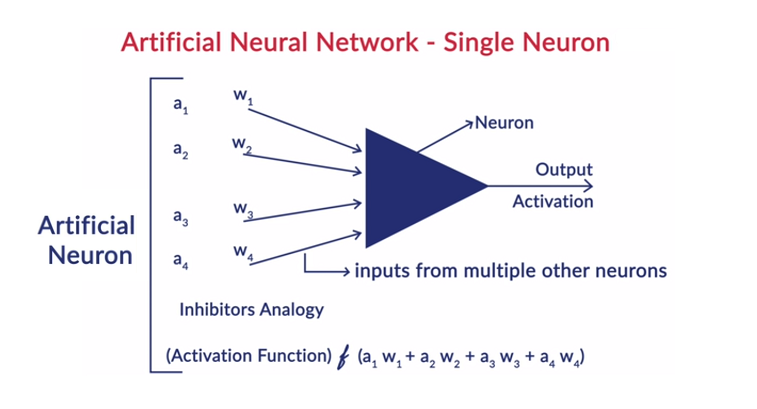## Assumptions in the Neural Networks

1. The neurons are arranged in the form of layers and these layers are arranged in a sequentially manner.
2. There is no communication between the neurons that are within in the same layer.
3. The entry point of neural networks is the input layer (first layer) and the exit point of the same is the output layer (last layer).
4. Every interconnection in the neural network has some weight associated with it and every neuron has a bias associated with it.
5. Same activation function is applied to all the neurons in a certain layer.

## Different Deep Learning Algorithms

1. Fully Connected Neural Network

In Fully Connected Neural Network (FCNNs), each neuron in one layer is connected to every other neuron in the next layer. These layers are referred to as Dense layers for the very same reason. These layers are very expensive computationally as every neuron connects with all the other neurons.

It is preferred to use this algorithm when the number of neurons in the layers are less, otherwise it would require a lot of computational power and time to perform the operations. It may also lead to overfitting due to its full connectivity.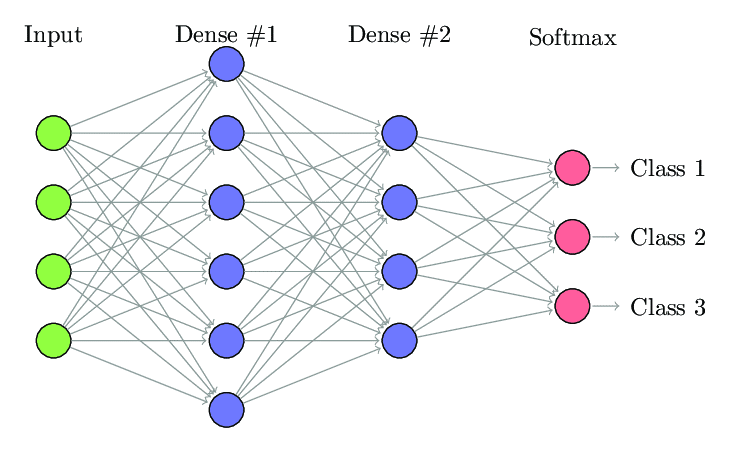Fully Connected Neural Network (Source: Researchgate.net)

2. Convolutional Neural Network (CNNs)

The Convolutional Neural Network (CNNs) are a class of neural networks which are designed to work with the visual data. i.e. images and videos. Thus, it is used for many image processing tasks like Optical Character Recognition (OCR), Object Localization etc. CNNs can also be used for video, text, and audio recognition.

The images are made up of pixels that determine the intensity of the whiteness in the image. Each pixel of an image is a feature which will be fed to the neural network. For example, an 128×128 image indicates the image is made up of 16384 pixels or features. It will be fed as a vector of size 16384 to the neural network. For colour images, there are 3 channels (one for each – Red, Blue, Green). In that case, the same image in colour would be made up 128x128x3 pixels.

There is hierarchy in the layers of the CNN. The first layer tries to extract the raw features of the images like horizontal or vertical edges. The second layers extract more insights from the features that are extracted by the first layer. The subsequent layers would then dive deeper into the specifics to identify certain parts of an image such as hair, skin, nose etc. Finally, the last layer would classify the input image as human, cat, dog etc.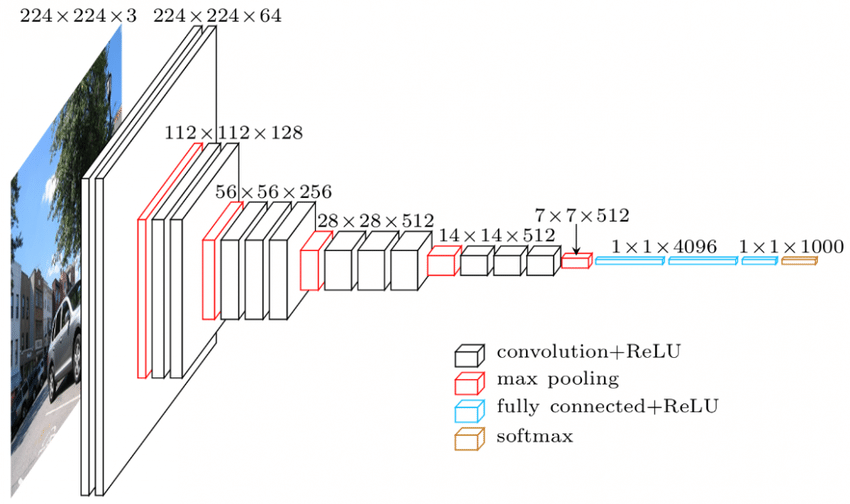Source

VGGNet Architecture – One of the widely used CNNs

There are three important terminologies in the CNNs:

• Convolutions – Convolutions is the summation of element wise product of the two matrices. One matrix is a part of input data and the other matrix is a filter which is used to extract features from the image.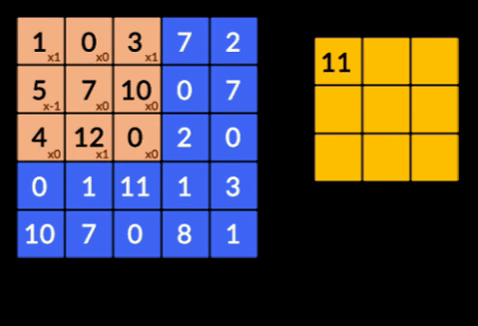• Pooling Layers – The aggregation of the extracted features is done by Pooling Layers. These layers generally compute an aggregate statistic (max, average etc) and makes the network invariant to the local transformations.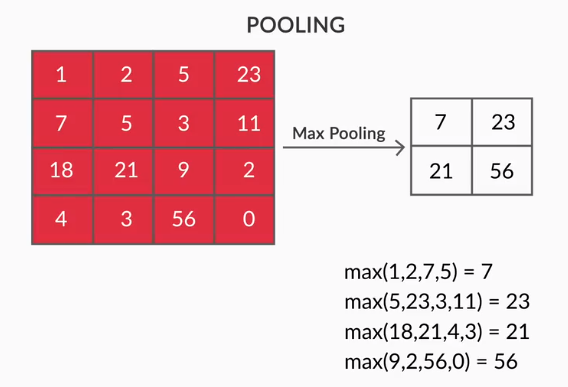• Feature Maps – A neuron is CNN is basically a filter whose weights are learnt during its training. Each neuron looks at a particular region in the input which is known as its receptive field. A Feature Map is a collection of such neurons which look at different regions of the image with same weights. All the neurons in a feature map try to extract same feature but from different regions of the image.

3. Recurrent Neural Networks (RNNs)

Recurrent Neural Networks are designed to deal with sequential data. Sequential data means data that has some connection with the previous data such as text (sequence of words, sentences etc) or videos (sequence of images), speech etc.

It is very important to understand the connection between these sequential entities, otherwise it would not make sense to jumble the whole paragraph and try to derive some meaning out of it. RNNs were designed to process these sequential entities. A good example of RNNs being used is the auto generation of subtitles in YouTube. It is nothing but Automatic Speech Recognition implemented using RNNs.

The main difference between the normal neural networks and recurrent neural networks is that the input data flows along two dimensions – time (along the length of the sequence to extract features out of it) and depth (normal neural layers). There are different types of RNNs and their structure changes accordingly.

• Many to One RNN: – In this architecture, the input fed to the network is a sequence and the output is a single entity. This architecture is used in tackling problems like sentiment classification or to predict the sentiment score of the input data (Regression problem). It can also be used to classify videos into certain categories.
• Many to Many RNN: – Both, the input and the output are sequences in this architecture. It can be further classified on the basis of the length of the input and output.
1. Same length: – The network produces an output at each timestep. There is a one to one correspondence between the input and output at each timestep. This architecture can be used as a part of speech tagger where each word of the sequence in the input is tagged with its part of speech as output at every timestep.
2. Different length: – In this case, the length of the input is not equal to the length of the output. One of the uses of this architecture is language translation. The length of a sentence in English can be different from the corresponding Hindi sentence.
• One to Many RNN: – The input here is a single entity whereas the output is a sequence. These kinds of neural networks are used for tasks like generation of music, images etc.
• One to One RNN: – It is a traditional neural network wherein the input and output are single entities.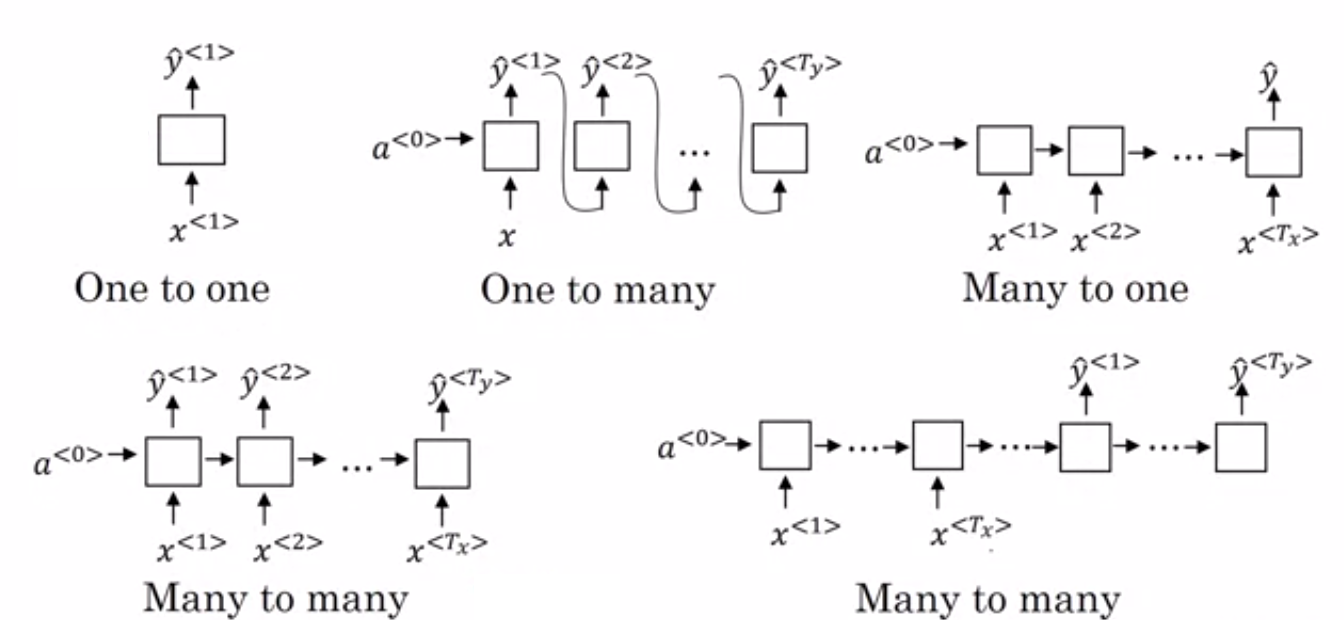Types of RNNs (Source: iq.opengenus.org)

4. Long – Short Term Memory Networks (LSTM)

One of the drawbacks of Recurrent Neural Networks is vanishing gradient problem. This problem is encountered when we are training neural networks with gradient-based learning methods like Stochastic gradient descent and backpropagation. The gradients of the activation function are responsible for updating the weights of the networks.

They become so small that it hardly affects the weights of the neural networks to change. This prevents the neural networks from training. RNNs face this issue when they are having difficulties in learning long term dependencies.

Long – Short Term Memory Networks (LSTM) were designed to encounter this very problem. LSTM consists of a memory unit which can store the information which is relevant to the previous information. Gated Recurrent Units (GRUs) are also a variant of RNNs that help in vanishing gradient problems.

Both use gating mechanism to solve this issue. GRU uses less training parameters and thus use less memory than LSTM. This enables GRUs to train faster but LSTM provide more accurate results where the input sequences are long.

Generative Adversarial Networks (GAN) is an unsupervised learning algorithm which automatically discovers and learns the patterns from the data. After learning these patterns, it generates new data as output which have the same characteristics as the input. It creates a model which is divided into two sub models – generator and discriminator.

The generator model tries to generate new images from the input whereas the role of discriminator model is to classify whether the data is a real image from the dataset or from the artificially generated images (images from the generated model).

The discriminator model generally acts as a binary classifier in form of convolutional neural network. With each iteration, both the models try to improve its results as the goal of generator model is to fool discriminator model in identifying the image and the goal of discriminator is to correctly identify the fake images.

6. Restricted Boltzmann Machine (RBM)

Restricted Boltzmann Machine (RBM) are non-deterministic neural networks with generative capabilities and learn the probability distribution over the input. They are restricted form of Boltzmann Machine, restricted in the terms of the interconnections among the nodes in the layer.

These involve only two layers i.e. visible layer and hidden layer. There is no output layer in the RBM and the layers are fully connected to each other. RBMs are now solemnly used as they have been replaced by the GANs. Multiple RBMs can also be put together to create a new network which can be tuned using gradient descent and backpropagation like the other neural networks. Such networks are called as Deep Belief Networks.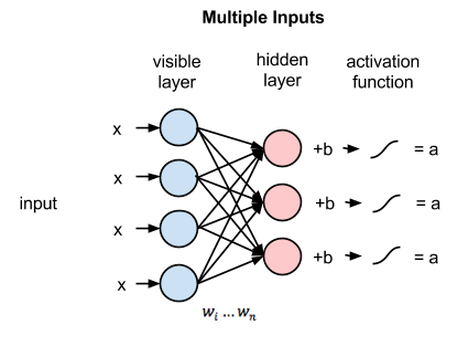Restricted Boltzmann Machine (Source: Medium)

7. Transformers

Transformers are a type of neural network architecture which were designed for neural machine translation. They involve an attention mechanism that focuses on a part of the information provided to the network. It involves two parts: Encoders and Decoders.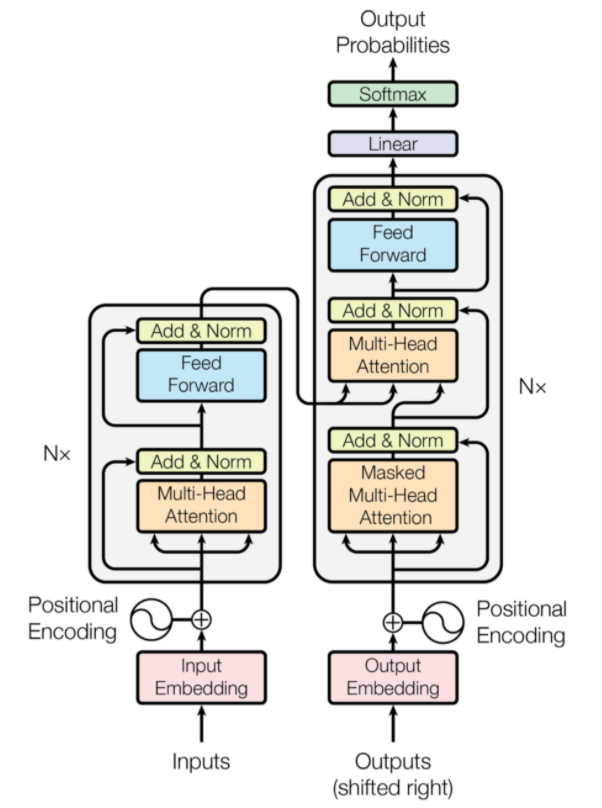Transformer Architecture (Source: arxiv.org)

The left part of the figure is the Encoder, and the right part is Decoder. The encoder and decoder can consist of multiple modules which can be stacked on the top of each other. The same is conveyed by Nx in the figure. The function of each encoder layer is to figure out which parts of the input are relevant to each other which are termed as encodings.

These encodings are then passed on to the next encoder layer as inputs. The decoder layer takes these encodings and processes them to generate the output sequence. The attentive mechanism weighs the significance of every other input and extracts information from these relationships to predict the output sequence. The encoder and decoder layers also consist of feed forward layers which are used for the further processing of the outputs.

8. Radial Basis Function Networks (RBFNs)

RBFN is one of the feedforward deep learning neural networks that use radial basis as their activation functions. They comprise three layers – input, hidden and output layer. RBFNs are usually employed for time-series prediction, classification, and regression.

RBFNs carry out these tasks by using the input vector to add the data into the input layer, confirming the identification and delivering the result by analyzing previous data sets. Sensitive neurons, present in the hidden layer, and nodes in the input layer allow for smooth classification of the data. The hidden layer has Gaussian transfer functions, which are inversely related to the distance between the output and the center of the neuron. The output layer incorporates linear combinations of the radial-based data, with the Gaussian functions being utilized as parameters within the neuron to generate the output.

## Popular AI and ML Blogs & Free Courses

 IoT: History, Present & Future Machine Learning Tutorial: Learn ML What is Algorithm? Simple & Easy Robotics Engineer Salary in India : All Roles A Day in the Life of a Machine Learning Engineer: What do they do? What is IoT (Internet of Things) Permutation vs Combination: Difference between Permutation and Combination Top 7 Trends in Artificial Intelligence & Machine Learning Machine Learning with R: Everything You Need to Know AI & ML Free Courses Introduction to NLP Fundamentals of Deep Learning of Neural Networks Linear Regression: Step by Step Guide Artificial Intelligence in the Real World Introduction to Tableau Case Study using Python, SQL and Tableau

9. Multilayer Perceptrons (MLPs)

MLP is a type of deep learning algorithm that also belongs to the feedforward approach incorporating several layers of perceptrons with activation functions. It consists of two fully connected layers – input and output, which are the same in number. However, they can also have multiple hidden layers. MLPs use these layers to build machine-translation software, image recognition and speech recognition.

The data is introduced to the input layer, after which a graph of neurons is formed in this layer, establishing a one-directional connection. The weight of the data exists only between the input and the hidden layer. MLPs then use the activation functions, such as the tanh function, sigmoid and ReLUs, to assess the nodes that are ready to fire. The primary objective of MLPs is to train models to comprehend the correlation between layers, ultimately resulting in the desired output from a given dataset.

10. Deep Belief Networks (DBNs)

DBNs contain several layers of stochastic gradient descent in deep learning as well as latent variables; this is why they are called generative models. Since the latent variable has binary values, it is referred to as a hidden unit.

Another name for DBNs is Boltzmann Machines due to the stacked Restricted Boltzmann Machine (RBM) layers, which enable communication between adjacent layers. Image, video recognition and motion capture are some uses of the DBNs.

Greedy algorithms power DBNs, with the most common approach being a layer-to-layer learning method via a top-down approach to generate weights. DBNs utilize a step-by-step Gibbs sampling approach on the top two hidden layers. These stages then sample from the visible units using an ancestral sampling method model. DBNs learn from latent values present in each layer, following a bottom-up pass approach.

Also Read: Deep Learning vs Neural Networks

## Conclusion

The article gave a brief introduction to the Deep Learning domain, the components used in the neural networks, the idea of deep learning algorithms, assumptions made to simplify the neural networks, etc. This article provides a restricted list of deep learning algorithms as there are a lot of different algorithms which are constantly being created to overcome the limitations of existing algorithms.

Deep Learning algorithms have revolutionized the way of processing videos, images, text etc. and they can be easily implemented by importing the required packages. Lastly, for all the Deep Learners, Infinity is the limit.

If you’re interested to learn more about deep learning techniquesmachine learning, check out IIIT-B & upGrad’s PG Certification in Machine Learning & Deep Learning which is designed for working professionals and offers 240+ hours of rigorous training, 5+ case studies & assignments, IIIT-B Alumni status & job assistance with top firms.Blog Author
Director of Engineering @ upGrad. Motivated to leverage technology to solve problems. Seasoned leader for startups and fast moving orgs. Working on solving problems of scale and long term technology strategy.
Get Free Consultation

Select CourseSelectBy clicking 'Submit' you Agree to

#### Our Popular Machine Learning Course

1Difference between CNN and ANN?

Artificial Neural Networks (ANNs) construct network layers parallel to the human neural layers: input, hidden, and output decision layers. ANNs are perceptive of faults and update themselves by restructuring themselves after a shortcoming. Convolutional Neural Networks (CNNs) are mainly image input focused. In CNNs, the first layer extracts the raw image. The next layer peers into the information found in the previous layer. The third layer identifies features of the image, and the final layer recognises the image. CNNs don’t require explicit input descriptions; They recognise data using spatial features. They are highly preferred for visual recognition tasks.

2Is Deep Learning providing an edge in Artificial Intelligence?

Artificial Intelligence (AI) has made technology more accurate and representative of the world. As a part of Machine Learning in AI, Deep Learning can efficiently process large amounts of data. It has a point to point approach for solving issues. Deep Learning has created efficient and quick systems, while Machine Learning systems have several steps to get started. Although Deep Learning needs a lot of training time, its testing reciprocity is instantaneous. Deep Learning is undeniably an integral part of Artificial Intelligence and has contributed to detecting auditory and visual data. It has made automated voice assistant devices, vehicles, and many other technologies possible.

3What are the limitations of Deep Learning?

Deep Learning has made strides in machine-human interaction and made technology serviceable for humankind in many ways. It has hurdles of extensive training, expensive equipment requirements, and large data prerequisites. It provides automated solutions, but it makes decisions that are not clear until the computation of numerous algorithms and neural networks is carried out. The pathway is traced back to the specific nodes, which is almost impossible; Machine Learning has a straight path of tracking processes and is preferable. Deep Learning does have many limitations, but its advantages outweigh them all.

## Suggested Blogs

91330
AWS Projects & Topics Looking for AWS project ideas? Then you’ve come to the right place because, in this article, we’ve shared multiple AWS proj